Hover over each button to see an explanation or play the video tutorials.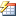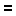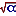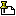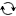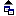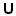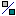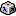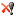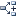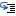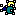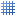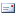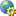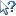When Auto XLC is on it monitors changes to your spreadsheet and redraws equations immediately. Thus worksheet formulas and XLC names and equations will always be up to date. However if you are working with very large datasheets it may slow you down. In this case you should switch off Auto XLC and manually refresh equations using XLC redraw buttons. Auto XLC will switch itself off if it takes longer that the XLC dwell time (this is changed using the XLC Settings button).Name the adjacent right cell for use in XLC equations. The name button also includes some XLC text shortcuts to easily produce Greek characters and subscripts. Any character preceded by ‘|’ will be converted to its equivalent Greek character. All characters after the first will be automatically subscripted and it will end with ‘ =‘. Note: if you remove the ‘ ‘ character before the final ‘=‘ automatic subscripting will not be enforced.XLC searches to the left of the current selection for cells containing formulas. XLC draws a mathematical equation using XLC Names directly from cell formulas. Wherever XLC inserts an equation it will also insert an EQS( ) function with a reference to the cell formula being made into an equation and some display settings. Remember to use OVD( )/UND( ) functions in cell formulas to tell XLC what to display OVer the Division line and UNder the Division line.Use table name tags so that XLC can ‘name’ cells lying within a table. Name each column using XLC’s ‘Name Equation’ button and use table tags to refer to the row position. XLC will identify any cell in the table using the column name and row table tag.Equations can be shown in an algebraic form or a numeric form. Note: use the ‘Clone Equation’ button if you wish to display in both forms.Copy an equation located above the selected cell and change the form of the equation from algebraic to numeric and vice-versa.Superscript any number within a text string so that units are quickly formatted.XLC uses its OVD( )/UND( ) functions to display OVer and UNder the equation Division line. You will use this construct a lot so copy it to the clipboard and paste into cell formulas quickly.Display a table of Greek characters and their XLC shortcuts. Any character preceded by ‘|’ will be converted to its equivalent Greek character but if you can’t remember your Greek alphabet this table will be a useful reminder.Removing all XLC functions from the worksheet so that it can be read by any Excel user even if they do not have XLC installed. WARNING: when XLC functions are deleted equations become static graphic objects and will no longer update. It is advisable to take a copy of the XLC version before you use this button.Removes all equation graphics from the worksheet. Remember to use the redraw buttons to display the equations again.The default equation number prefix is ‘Eqn’ but you can set it using the ‘XLC Settings’ button. Equation numbers are not shown when the ‘Auto XLC’ button is on. Use this button to re-render all worksheet equations with the correct equation numbers. Equations are renumbered from left to right and then top to bottom.Use this button to re-render all worksheet equations without displaying equation numbers.Change the active cell through a range of values and watch as the worksheet updates. This is particularly effective if charts are used.Process text to produce mathematical notation quickly. Any character preceded by ‘|’ will be converted to its equivalent Greek character. Any character preceded by ‘_’ will be subscripted. All characters between square brackets will be subscripted. Any character preceded by ‘^’ will be superscripted.Selects all graphical objects on the worksheet. This is particularly useful for making sketches to group objects together without having to select each one individually. Note if you do not wish to include equations use the ‘Hide Equations’ button first.This only works with XY scatter Charts. The button toggles between automatic XY scaling (to fit the chart window) or an equal scale for the X and Y axis (square grid). A square grid is useful to use scatter charts to draw sketches.Search the ExcelCalcs site for template calculations.Donate a workbook to the ExcelCalcs repository. Publish your work on the ExcelCalcs website where your work will be appreciated by your fellow users. ExcelCalcs rewards any content used with free subscriptions.XLC help via tutorials on the ExcelCalcs website.Insert your XLC release code to configure XLC in the XLC Pro mode. Set program preferences like equation prefix, force display of multiplication signs, change multiplication character, algebraic or numeric equations, vertical alignment, amazon site preference and Auto XLC dwell time (switch off automatic functionality if it is too slow).